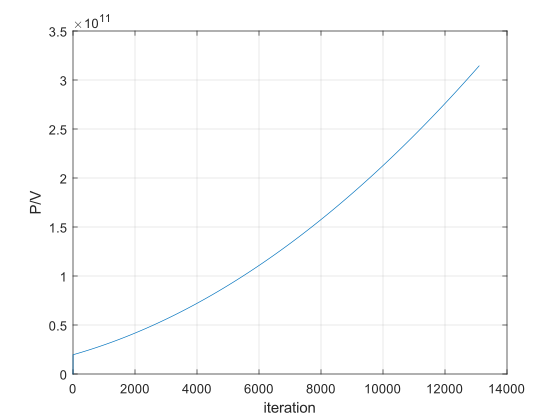# How to use a Loop to repeat two equations using the previous answers as the new variables.

4 views (last 30 days)
Rahul Marwaha on 15 Nov 2020
Answered: Les Beckham on 15 Nov 2020
I want the script to find the pressure values (p1,2,3,4, etc ...) until the values reaches 400000. The script should take the calculated V_2 value and use it it to find the new V_3 value. It should then use this value to find P3, this operation should continue until the P value is equal to 400000. I've tried many different loops but can't get it to repeat both equaiotns using the previous answer as the new variable.
% Piston Compressor Script
% First we define the non-varying values
V = 0.03; % Volume of air in tyre (m3)
T = 300; % time to inflate in seconds
P1 = 100000; % Atmospheric pressure (Pa)
% We can now compute a the cylinder volume
S = 0.02; % Stroke
B = 0.018; % Bore
Vc = pi/4 * B^2 * S;
% We now calculate the inital compressed pressure
P2 = P1 * ((V+Vc)/V)^1.35;
% We can now get the script to find the next pressures up to 4 bar
V_2 = (P1*(Vc))/P2;
P3 = P2 * ((V_2+V)/V)^1.35;
% Now need to repeat until P = 400000
V_3 = (P1*(Vc))/P3;
P4 = P3 * ((V_3+V)/V)^1.35;
% E.g next value would be
V_4 = (P1*(Vc))/P4;
P5 = P3 * ((V_4+V)/V)^1.35;

Les Beckham on 15 Nov 2020
This shows how to do the iteration using indexing and a loop instead of typing out each iteration manually:
I'm not sure that this gives the right answer as the volume calculation
V(idx) = P0*Vc / P(idx);
based on your original
V_2 = (P1*(Vc))/P2;
doesn't seem to include the 'volume of air in the tyre' which I called V0.
After the first iteration, the pressure volume relationship seems reasonable, though (see plot below).You should be able to adapt this to make necessary corrections.
% Piston Compressor Script
% First we define the non-varying values
V0 = 0.03; % Volume of air in tyre (m3)
T = 300; % time to inflate in seconds
P0 = 100000; % Atmospheric pressure (Pa)
% We can now compute the cylinder volume
S = 0.02; % Stroke
B = 0.018; % Bore
Vc = pi/4 * B^2 * S;
% We now calculate the inital compressed pressure
P(1) = P0;
P(2) = P0 * ((V0 + Vc) / V0)^1.35;
V(1) = V0;
% Iterate until P >= 400000
idx = 2;
while P(end) < 400000
V(idx) = P0*Vc / P(idx); %#ok<SAGROW>
P(idx+1) = P(idx) * ((V(idx) + V0) / V0)^1.35;
idx = idx + 1;
end
% Add the last value for V so that P and V are the same length
V(end+1) = P0*Vc / P(end);

### Community Treasure Hunt

Find the treasures in MATLAB Central and discover how the community can help you!

Start Hunting!